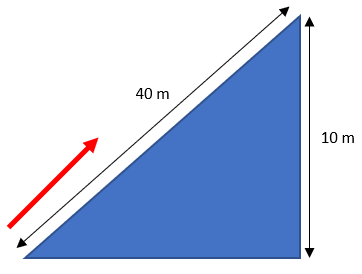## Pushing a Boulder

In the figure below, a 500 kg boulder is pushed 40 m along a slope. What is the potential energy at the top?Hint
Vertical distances are only relevant in potential energy problems. We can ignore the slope and angle.
Hint 2
Potential Energy in a Gravity Field:
$$U=mgh$$$where $$m$$ is the mass, $$g$$ is the acceleration due to gravity, and $$h$$ is the height. Vertical distances are only relevant in potential energy problems. We can ignore the slope and angle. For Potential Energy in a Gravity Field: $$U=mgh$$$
where $$m$$ is the mass, $$g$$ is the acceleration due to gravity, and $$h$$ is the height. Thus,
$$U=(500kg)(9.8m/s^2)(10m)=49,000\frac{kg\cdot m^2}{s^2}=49\:kJ$$\$
49 kJ